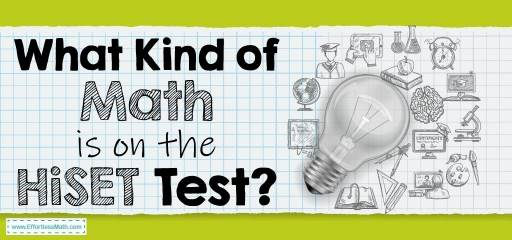# What Kind of Math Is on the HiSET Test?The High School Equivalency Test (HiSET), commonly known as HiSET, is an alternative to the GED test. This test has been published since 2014 by the ITP (Iowa Testing Programs) and ETS (Educational Testing Service) for those who have failed to complete high school to obtain a diploma and achieve a university education. Bypassing this test, students can obtain a high school equivalency certificate issued by their state.

HiSET test takers can participate in the test by computer or paper and pencil. The HiSET test has the following 5 sections:

• Social Studies
• Language Arts Writing
• Science
• Mathematics

The HiSET Mathematics test is a 90-minute, single-section test that covers basic mathematics topics, quantitative problem-solving, and algebraic questions. There are approximately 50-55 Multiple-choice questions in the Mathematics section. The calculator is allowed in the Math section.

## Algebraic Concepts

Test takers should note that the algebra section is the most important part of the HiSET Math test. Algebraic concepts make up the bulk of test questions. In this section, test takers deal with expressions and formulas and must know how to answer to a variety of equations and how to interpret functions. It is also important to know how to use graphs to solve equations.

## Numbers and Operations on Numbers

In this part of the HiSET Math test, it is important to know how to work with numbers in different ways, from rounding them to turning them into another format. In general, in this section, you can find questions about averages, exponents, recreate expressions, and scientific notation. You should be able to recreate expressions and solve questions using your knowledge and applying operations on different types of numbers.

## Data Analysis, Probability, and Statistics

In this section, you should be able to solve problems using statistical data. You should decipher the information in different ways. You are also expected to be able to find the average of a data set and then use it to solve population problems. Knowing how to solve problems related to data sets and basic probabilities related to different events in this section is very important.

## Measurement and Geometry

In the Measurement and Geometry section, you will be asked to calculate the size and similar qualities of shapes using the available information. To succeed in this field, you must be well acquainted with all geometric shapes and how to calculate their volume and area. You also need to know the Pythagorean theorem and be able to use it properly when needed.

## FAQs:

### Is the HiSET Math test hard?

HiSET Math test, like any other test, can be challenging. However, if you are well prepared to answer the HiSET Math questions, you will have no problem succeeding in this test.

### How do I study for the HiSET Math?

How you prepare for the HiSET math test is very important. You can use good prep books to prepare well for the HiSET Math test. You can also use online practice tests for further practice after studying the HiSET exam courses.

### Can I bring a calculator to the HiSET test?

No, you cannot bring a calculator to the HiSET test session, but the test center will provide you with a calculator.

### How is the HiSET Test Scored?

Each question is assigned one point which is added to your score with each correct answer. The math section has a maximum of 20 points. for more information, you can check here.

## The Best Books to Ace the HiSET Math Test

### What people say about "What Kind of Math Is on the HiSET Test? - Effortless Math: We Help Students Learn to LOVE Mathematics"?

No one replied yet.

X
52% OFF

Limited time only!

Save Over 52%

SAVE \$40

It was \$76.99 now it is \$36.99